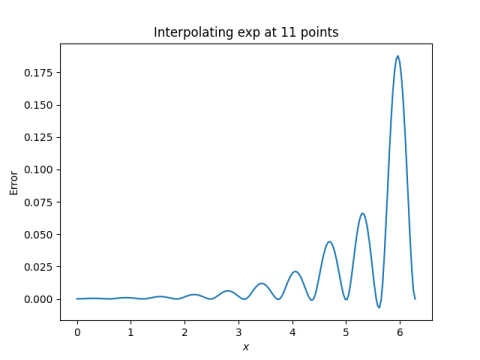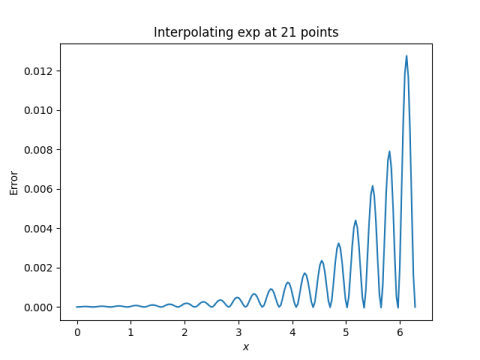# Error estimates for splines with more boundary conditions

Yesterday I wrote about rates of convergence for natural cubic splines. This brief post reports similar results for more boundary conditions.

As explained in the earlier post, a cubic spline that interpolates a function f at n+1 points satisfies 4n -2 equations in 4n variables. Two more equations are necessary to uniquely determine the interpolating cubic spline.

A natural cubic spline S over [a, b] satisfies

S”(a) = 0
S”(b) = 0.

There are other ways of coming up with two more equations. You might match first derivatives at the end points

S‘(a) = f‘(a)
S‘(b) = f‘(b)

or second derivatives

S”(a) = f”(a)
S”(b) = f”(b).

Or if f is periodic you could require first and second derivatives of the spline to match at a and b.

S‘(a) = S‘(b)
S”(a) = S”(b).

In each case, you get the same order of convergence as we reported earlier for the natural cubic spline, assuming f is 4 times continuously differentiable over [a, b]. The error goes down like O(1/n4).

Source: D. Kershaw. A Note on the Convergence of Interpolatory Cubic Splines. SIAM Journal on Numerical Analysis, Mar., 1971, Vol. 8, No. 1 pp. 67-74.

## Example

When we use natural cubic splines to fit exp(x) over [0, 2π] in the earlier post, the error was fairly large. We get better results when we specify the derivatives at the two ends of the interval. Here’s what we get for 11 points:The error is about 7 times smaller than with natural cubic splines.

And here’s what we get for 21 points:In this case the error is about 8x smaller than with natural cubic splines.

When we go from 11 to 21 points, specifying the derivatives on each end, the error drops by about a factor of 15, close value of 16 we’d expect in the limit.

Incidentally, to specify the boundary conditions of the spline in Python we need to add an option parameter `bc_type` in the call to `CubicSpline`. Otherwise the code is the same as before. In our example

`    bc_type=((1, 1), (1, np.exp(2*np.pi)))`

We set `bc_type` equal to a pair of pairs. The first pair gives a boundary condition on the left end and the second gives a boundary condition on the right. The first argument of the inner pair in both cases is 1, because we’re specifying 1st derivatives. Use a 2 to specify 2nd derivatives. The second argument to the pair the value of the derivative: exp(0) = 1 on the left, and exp(2π) on the right.